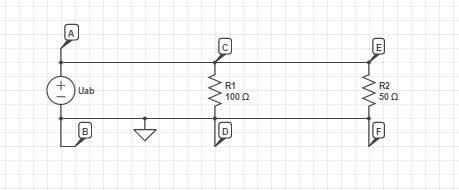# A question about voltage between two points in a circuit

• Engineering

## Homework StatementThis is a simple circuit diagram from the class were we derived the equations for the equivalent resistance of parallel resistor connection. I have a quick question about this though..
Is the voltage between points ##A,B## the same as voltage between points ##C,D## and ##E,F##?

## Homework Equations

3. The Attempt at a Solution [/B]
Can someone convince me that they are in fact the same voltages cause even though we said they were i keep imagining that the current which divides at point ##C## and goes through the resistor ##R_1## makes a different voltage than the original and the same goes for the other part. What should i be realizing?

## Answers and Replies

NascentOxygen
Staff Emeritus
Science Advisor
Is the voltage between points ##A,B## the same as voltage between points ##C,D## and ##E,F##?
These potential differences are all equal. The basis for saying this is that the conductive "wire" joining points A, C and E is ideal or approximately ideal, i.e., it has zero Ohms resistance. So regardless of how much current that wire carries, there is no significant voltage drop along it. The same applies to the conductor joining B, D and F.

•diredragon
For the purposes of the question the voltages are all the same but if you had a sensitive enough voltmeter, a 4 point probe to be exact, you would see slight differences in the voltages all up and down the wires but the changes would be in the microvolt or maybe millivolt difference because although for the purposes of the question the wire is considered zero ohms, nothing short of a superconductor has in fact zero ohms. It might be 10 milliohms or some such but because the resistance is NOT exactly zero there will be voltage differences along the wires. But not enough to change the answer to the question.,